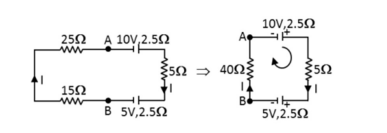Question

# The potential difference between and in the circuit shown in figure will beA
B
C
D
Hard
SolutionVerified by Toppr
Correct option is D)

## Hint: Kirchhoff’s second law, also known as the Kirchhoff’s voltage law (KVL) states that the sum of all voltages around a closed loop in any circuit must be equal to zero.Step 1: To find the e.m.f of the circuitThe resultant resistance of circuit is     The resultant e.m.f of the circuit is given by In closed loop According kirchhoff's Second lawTherefore, the correct answer is D is .00